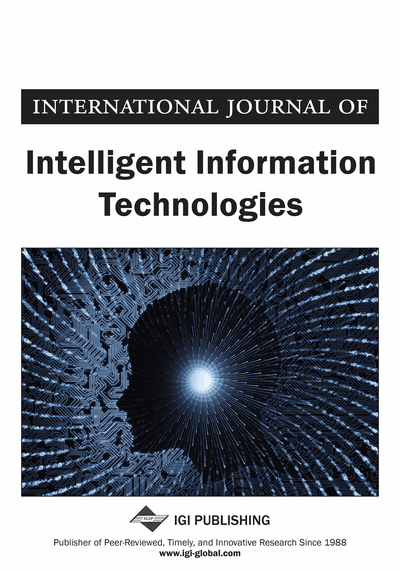# An Intelligent Operator for Genetic Fuzzy Rule Based System

C. Rani (Anna University of Technology Coimbatore, India) and S. N. Deepa (Anna University of Technology Coimbatore, India)
DOI: 10.4018/jiit.2011070103

## Abstract

This paper proposes a modified form of operator based on Particle Swarm Optimization (PSO) for designing Genetic Fuzzy Rule Based System (GFRBS). The usual procedure of velocity updating in PSO is modified by calculating the velocity using chromosome’s individual best value and global best value based on an updating probability without considering the inertia weight, old velocity and constriction factors. This kind of calculation brings intelligent information sharing mechanism and memory capability to Genetic Algorithm (GA) and can be easily implemented along with other genetic operators. The performance of the proposed operator is evaluated using ten publicly available bench mark data sets. Simulation results show that the proposed operator introduces new material into the population, thereby allows faster and more accurate convergence without struck into a local optima. Statistical analysis of the experimental results shows that the proposed operator produces a classifier model with minimum number of rules and higher classification accuracy.
Article Preview
Top

## 1. Introduction

Fuzzy Logic has been successfully applied to many control (Gestwa et al., 2003), modeling (Meziane et al., 2007), and classification problems (Pedrycz et al., 2009). The key to the success of the fuzzy logic is its ability to incorporate human expert knowledge. An important issue in the design of fuzzy logic based classifier model is the formation of if-then rules and the membership functions. In general, the rules and membership functions are formed from the experience of the human experts. For the problems with many input variables, the possible number of rules (Ali et al., 2011) increases exponentially, which makes it difficult for experts to define a complete rule set for good system performance.

Data-driven approaches (Wang et al., 1992) have been proposed for developing the fuzzy classifier model from numerical data without domain experts. Abe et al. (1995) proposed a rule generation method in which each fuzzy if-then rule was represented by a hyper box in multidimensional pattern space. This approach is very weak in self learning and determining the required number of fuzzy if-then rules. Ishibuchi et al. (1996) proposed a heuristic method for generating fuzzy if-then rules for pattern classification problems using grid-type fuzzy partition. In this method, a prior knowledge on linguistic values is required for specifying the membership function. Also, this method fails to handle high dimensional problems due to curse of dimensionality.

Genetic Fuzzy Rule Based System (GFRBS) (Cordon et al., 2004) is one such approach in which a fuzzy classifier model is augmented by a learning process based on Genetic Algorithm (GA). GA (Goldberg, 1989; Tripathi et al., 2011) is a search algorithm based on the mechanics of natural genetics. The GFRBS proposed in the literature falls into four categories: (1) Learning fuzzy rules with fixed fuzzy membership functions (Ishibuchi et al., 1999) (2) Learning fuzzy membership functions with fixed fuzzy rules (Yuhui et al., 1999), (3) Learning fuzzy rules and membership functions in stages (Setnes et al., 2000) and (4) Learning fuzzy rules and membership functions simultaneously (Russo, 2000). This paper follows the last approach.

Representation of solution variables and genetic operations are the most important issues in designing a fuzzy system using GA. Ishibuchi et al. (1995) coded a set of fuzzy if-then rules using binary string and treated as an individual in genetic algorithm. In Wang et al., (1998) each fuzzy rule set with its associated membership function is first transformed into an intermediary representation and then further encoded as a binary string. Integer string was used in (Yuhui et al., 1999) to represent the rule set and the membership function. Real-coded GA (Roubos et al., 2001) is applied for the simultaneous optimization of the parameters of the antecedent membership functions and the rule consequents. In this paper a mixed form of representation is followed which represents the rule set using integer number and the values of membership function using floating point numbers.

## Complete Article List

Search this Journal:
Reset
Open Access Articles: Forthcoming
Volume 16: 4 Issues (2020): 2 Released, 2 Forthcoming
Volume 15: 4 Issues (2019)
Volume 14: 4 Issues (2018)
Volume 13: 4 Issues (2017)
Volume 12: 4 Issues (2016)
Volume 11: 4 Issues (2015)
Volume 10: 4 Issues (2014)
Volume 9: 4 Issues (2013)
Volume 8: 4 Issues (2012)
Volume 7: 4 Issues (2011)
Volume 6: 4 Issues (2010)
Volume 5: 4 Issues (2009)
Volume 4: 4 Issues (2008)
Volume 3: 4 Issues (2007)
Volume 2: 4 Issues (2006)
Volume 1: 4 Issues (2005)
View Complete Journal Contents Listing